These extraordinary second grade worksheets take skills mastered in first grade to the next level. Addition and subtraction become more exciting with 2-and 3-digit problems. Students will be thrilled to learn the new topics of multiplication and division in appealing ways. Other fundamental skills include coins, money, time, patterns, measurement, basic fractions, and even algebra. Skills can be chosen for dynamic worksheets that are customized for students' abilities.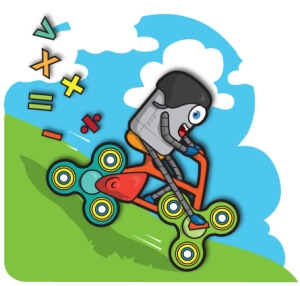Monthly Math Challenge Workbooks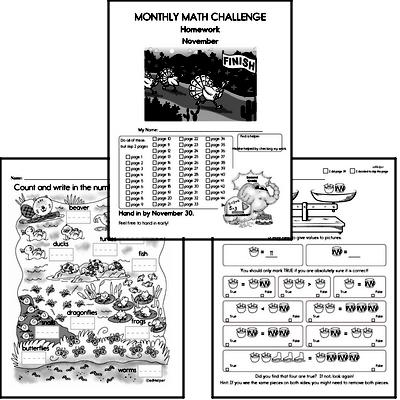Mental Math Workbooks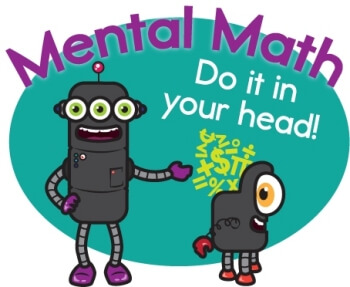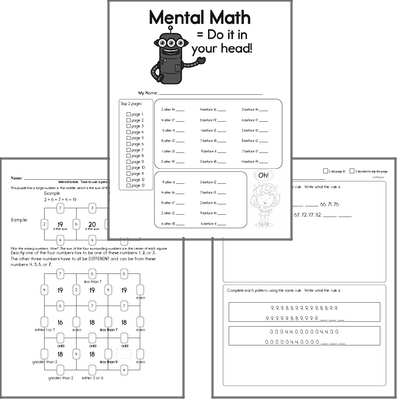Mental Math Workbooks

Second Grade Math Worksheets Activity Workbook - No Prep and Not Boring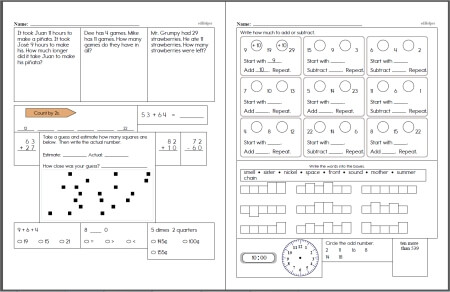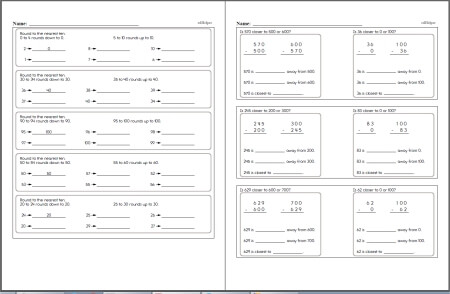Second Grade Math Book New Second Grade Math Workbook Created Each book contains word problems, mixed math, and other second grade review materials. Use it each week for daily practice in your classroom.

Daily Second Grade Math and Critical Thinking Practice

Second Grade Daily Homework and Practice Pages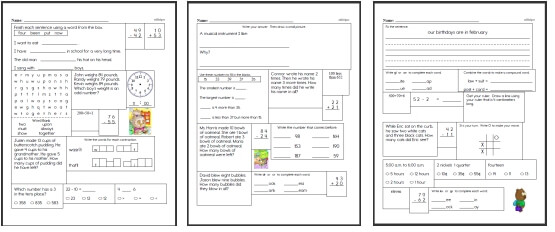Second Grade Daily Homework

Level 1: Easiest (First 2 months of school)
Level 2: Next 3 months of school
Level 3: Next 3 months of school
Level 4: Entire Grade (Final months of school)

Weekly Word Problems
Last week's second grade math word problems
This week's second grade math word problems
Next week's second grade math word problems
Daily Review: Customize math review skills with word problems, language arts, and science

Runaway Math Puzzles
 Second grade runaway math puzzles Runaway Math Puzzles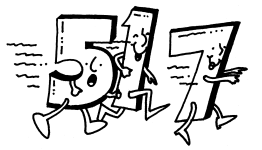Counting

Number Lines

Comparing Numbers

Hundreds Chart Pieces Puzzle

Ordering Numbers
Numbers: 0-10
Fill in the missing numbers (Just before, after, and between)
Greater than, less than, and equal to (circle the words)
Circle the larger number
Circle the smaller number
Order 3 numbers (from smallest to largest)
Order 3 numbers (from largest to smallest)
Order 4 numbers
Ordering Mixed Review

Numbers: 11-20
Fill in the missing numbers (Just before, after, and between)
Greater than, less than, and equal to (circle the words)
Circle the larger number
Circle the smaller number
Order 3 numbers (from smallest to largest)
Order 3 numbers (from largest to smallest)
Order 4 numbers
Ordering Mixed Review

Numbers: 0 to 20
Fill in the missing numbers (Just before, after, and between)
Greater than, less than, and equal to (circle the words)
Circle the larger number
Circle the smaller number
Order 3 numbers (from smallest to largest)
Order 3 numbers (from largest to smallest)
Order 4 numbers
Ordering Mixed Review

Numbers: 0 to 50
Fill in the missing numbers (Just before, after, and between)
Greater than, less than, and equal to (circle the words)
Circle the larger number
Circle the smaller number
Order 3 numbers (from smallest to largest)
Order 3 numbers (from largest to smallest)
Order 4 numbers
Ordering Mixed Review

Numbers: 0 to 99
Fill in the missing numbers (Just before, after, and between)
Greater than, less than, and equal to (circle the words)
Circle the larger number
Circle the smaller number
Order 3 numbers (from smallest to largest)
Order 3 numbers (from largest to smallest)
Order 4 numbers
Ordering Mixed Review

Ordering and Counting
 Mazes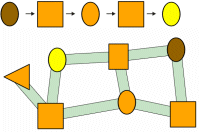Number Mazes and Shapes Mazes

 Matching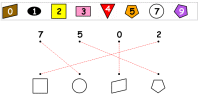Matching Shapes, Numbers, and Colors

 Combined Shapes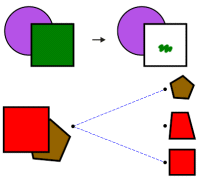Combined Shapes: Coloring and Matching

 Ordering Animals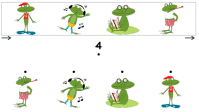Ordering Animals

 Ordering Shapes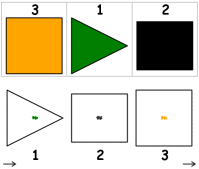Ordering Shapes
 Grids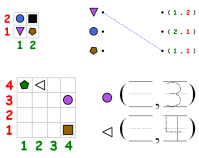Grids: Shapes, Positions, Writing Numbers

 Greater and Less Than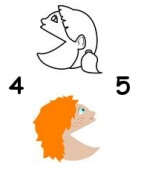Greater and Less Than

Place Value

Place Value to 99: Ones and Tens
Numbers 11 to 49
Graphics: count and write the number of ones and tens
Given a number: write the number of ones and tens
Given ones and tens: write the number
Fill in the missing number
Circle the larger number
Circle the smaller number

Numbers 51 to 99
Graphics: count and write the number of ones and tens
Given a number: write the number of ones and tens
Given ones and tens: write the number
Fill in the missing number
Circle the larger number
Circle the smaller number

Numbers 1 to 99
Graphics: count and write the number of ones and tens
Given a number: write the number of ones and tens
Given ones and tens: write the number
Fill in the missing number
Circle the larger number
Circle the smaller number

Review
Place Value to 99: Ones and Tens Review

Odd and Even Numbers

Number Patterns
Even and Odd Numbers
Circle odd or even (numbers 1 to 29)
Circle odd or even (numbers 1 to 99)
Write odd or even

Before, After, Between
Just before and after, Just before, Just after
Even and odd numbers: numbers 3 to 29 (easier)
Even and odd numbers: numbers 3 to 29
Even and odd numbers: numbers 10 to 99 (easier)
Even and odd numbers: numbers 10 to 99

Counting
Counting forward and backward (with a number line)
Counting forward and backward

Skip-counting (includes hint on how to skip count)
Skip count by 2's (numbers 2 to 49)
Skip count by 3's (numbers 2 to 49)
Skip count by 4's (numbers 2 to 49)
Skip count by 5's (numbers 2 to 49)
Skip count by 2's, 3's, 4's, and 5's (numbers 2 to 49)
Skip count by 2's (numbers 2 to 99)
Skip count by 3's (numbers 2 to 99)
Skip count by 4's (numbers 2 to 99)
Skip count by 5's (numbers 2 to 99)
Skip count by 2's, 3's, 4's, and 5's (numbers 2 to 99)
Mix of skip count with larger problems and more blanks

Skip-counting
Mix of complete the pattern (numbers 2 to 49)
Mix of complete the pattern (numbers 2 to 99)
Mix of complete the pattern (numbers 2 to 99 with more blanks per question)

Repeating Patterns
One blank at end (numbers 1-20)
One blank at end (numbers 1-99)
Two to three blanks at end (numbers 1-20)
Two to three blanks at end (numbers 1-99)
One blank anywhere in the pattern (numbers 1-99)

Problem Solving
Problem solving

Review
Number Patterns Mixed Review

Subtraction and Introduction to Subtraction

Blocks (black and white)
Find sum (2-7 blocks)
Fill in the missing number (2-7 blocks)
Complete the addition fact (2-7 blocks)
Find sum (6-9 blocks)
Fill in the missing number (6-9 blocks)
Complete the addition fact (6-9 blocks)

Blocks (color)
Find sum (2-7 blocks)
Fill in the missing number (2-7 blocks)
Complete the addition fact (2-7 blocks)
Find sum (6-9 blocks)
Fill in the missing number (6-9 blocks)
Complete the addition fact (6-9 blocks)

Dominoes
Fill in the blanks (sum of 2-9)
Complete the addition fact (sum of 2-9)
Fill in the blanks (sum of 6-12)
Complete the addition fact (sum of 6-12)

Fill in the missing numbers

Word search: adding the number 1
Word search: adding the number 2
Word search: adding the number 3

Word problems and review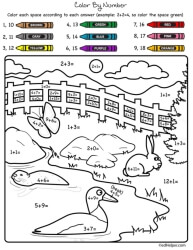Color by Number Addition

Number Bonds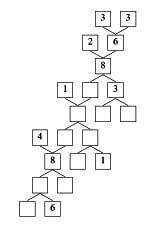Number Bonds

Math Facts

Addition Math Facts: Numbers 0 to 9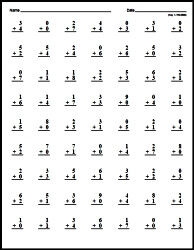Addition Facts: Numbers 0 to 9 Cross out the incorrect facts Make Custom Quick Math Facts Printable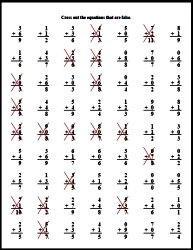Addition Facts: Focusing on a number

Counting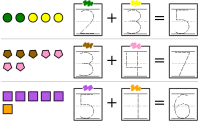Counting (part 1)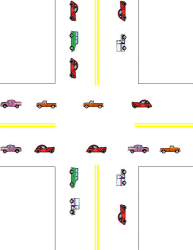Counting (part 2)

Subtraction: Numbers 0 to 10
Subtracting the number 1
Subtracting the number 2
Subtracting the number 3
Subtracting the number 4
Subtracting the number 5
Subtracting the number 6
Subtracting 7-10
Subtracting 1-2
Subtracting 2-4
Subtracting 2-5
Subtracting 1-9

Fill in the missing numbers
Subtracting 1-3
Subtracting 2-4
Subtracting 2-5
Subtracting 4-7
Subtracting 3-8
Subtracting 1-9

Find subtraction facts
Word search: subtract the number 1
Word search: subtract the number 2
Word search: subtract the number 3
Word search: subtract the number 4
Word search: subtract the number 5
Word Search: subtracting 1-3
Word Search: subtracting 3-5
Word Search: subtracting 4-9

Subtraction Math Facts: Numbers 0 to 9Subtraction Facts: Numbers 0 to 9 Cross out the incorrect facts Make Custom Quick Math Facts PrintableSubtraction Facts: Focusing on a number
Subtraction with the number 1
Subtraction with the number 2
Subtraction with the number 3
Subtraction with the number 4
Subtraction with the number 5
Subtraction with the number 6
Subtraction with the number 7
Subtraction with the number 8
Subtraction with the number 9

Addition with Blocks (includes the total block count)
Find the sum
Fill in one number

Find the sum
Fill in one number

Doubles and Doubles + 1
Mixed vertical and horizontal
Fill in the missing numbers
Word search

Blocks: find the sum
Blocks: fill in one number
Mixed vertical and horizontal

Addition with sums from 11 through 15
Fill in the missing numbers
Word search
Adding three numbers: vertical and horizontal
Adding three numbers: fill in the missing numbers
Word problems

Addition with sums from 11 through 19
Fill in the missing numbers
Word search
Adding three numbers: vertical and horizontal
Adding three numbers: fill in the missing numbers
Word problems

Review

Subtraction Through 20
Subtraction with Blocks: subtracting 1-5 (includes the answer)
Find the difference
Fill in one number
Complete the subtraction fact

Subtraction with Blocks: subtracting 1-5
Find the difference
Fill in one number
Complete the subtraction fact

Subtraction with Blocks: subtracting 6-10 (includes the answer)
Find the difference
Fill in one number
Complete the subtraction fact

Subtraction with Blocks: subtracting 6-10
Find the difference
Fill in one number
Complete the subtraction fact

Subtracting 1 to 4 from 10 to 19
Subtracting 5 to 9 from 10 to 19
1 addition and 2 subtraction facts
1 subtraction and 2 addition facts
Mixed

Subtracting from 16 through 20
Horizontal and vertical subtraction
Vertical subtraction
Horizontal subtraction
Fill in the missing numbers
Word search
Word problems

Fill in the missing numbers (number is same for each set)
Fill in the missing numbers

Subtracting from 11 through 20
Horizontal and vertical subtraction
Vertical subtraction
Horizontal subtraction
Fill in the missing numbers
Word search
Word problems

Review
Subtraction Through 20 Mixed Review

Addition and Subtraction Through 20 Review
Blocks
Find the difference
Fill in one number
Complete the subtraction fact

Mixed
Horizontal and vertical addition and subtraction
Fill in the missing numbers
Word search

Word Problems and Review
Addition and subtraction through 20 word problems
Addition and Subtraction Through 20 Mixed Review

Adding and Subtracting Two-Digit Numbers (No Regrouping)
Adding and Subtracting with one one-digit number and one two-digit number (No Regrouping)
Adding and subtracting tens and ones with blocks
Vertical addition and subtraction - 2 digits on top and 1 digit on bottom
Vertical addition and subtraction - 1 digit on top and 2 digits on bottom
Vertical addition and subtraction - 2 digits and 1 digit (mixed)
Fill in the missing number in each problem
Horizontal and vertical addition and subtraction
Fill in the missing numbers

Two-Digit Addition and Subtraction (No Regrouping)
Adding and subtracting tens and ones with blocks
Fill in the missing number in each problem
Horizontal and vertical addition and subtraction
Fill in the missing numbers

Word problems and review (No Regrouping)
Two-Digit addition and subtraction word problems
Two-Digit addition and subtraction Mixed Review (No Regrouping)

Data, Graphs, and Picture Graphs
Picture Graphs
Picture graphs and questions
Use the data to make a horizontal bar graph
Use the data to make a vertical bar graph
Table Graphs (with pictures)
Table graphs and questions
Use the data to make a horizontal bar graph
Use the data to make a vertical bar graph
Tally Charts
Tally charts and questions
Use the data to make a horizontal bar graph
Use the data to make a vertical bar graph
Mixed Review
Data, Graphs, and Picture Graphs Mixed Review

Introduction to 2-Digit Addition with Regrouping
Regroup a number (with blocks)
Regroup a number
Calculate a sum. Do you need to regroup?
Adding a 2-digit number to a 1-digit number
Introduction to 2-digit Addition with Regrouping Mixed Review

Adding 2-digits to 1-digit (all are regrouping)
Adding 2-digits to 1-digit (most are regrouping)
2-digit addition (has tens/ones but no carry box)
Fill in the missing digit
2-digit addition of cents (with carry box)
2-digit addition of cents (no carry box)
Problem solving
2-digit Addition with Regrouping Mixed Review

Introduction to 2-Digit Subtraction with Regrouping
Regroup a number (with blocks)
Regroup a number
Do you need to regroup? (includes a number line)
Do you need to regroup?
Subtract one digit from two digits and then add to check (includes numbers in addition formula)
Subtract one digit from two digits and then add to check
Subtract one digit from two digits and then add to check (includes problems with no regrouping)
Subtract one digit from two digits and then add to check (does not include "What is")
2-digit subtraction
2-digit subtraction and then add to check
2-digit subtraction with cents
2-digit subtraction
Problem solving (with subtraction equation)
Introduction to 2-digit Addition with Regrouping Mixed Review

2-Digit Subtraction with Regrouping
Subtract 1-digit from 2-digits
Fill in the missing number: 1-digit from 2-digits
Subtracting cents: 1-digit from 2-digits
Subtracting cents: 2-digit subtraction
2-digit subtraction
Fill in the missing number: 2-digit subtraction
Problem solving
2-digit Subtraction with Regrouping Mixed Review

2-Digit Addition and Subtraction with Regrouping
2-digit and 1-digit addition and subtraction (with tens/ones shown)
2-digit and 1-digit addition and subtraction (all are regrouping)
Fill in the missing numbers (all are regrouping)
Horizontal 2-digit and 1-digit addition and subtraction (all are regrouping)
Mixed 2-digit and 1-digit addition and subtraction (all are regrouping)
2-digit and 1-digit addition and subtraction (most are regrouping)
Fill in the missing numbers (most are regrouping)
Horizontal 2-digit and 1-digit addition and subtraction (most are regrouping)
Mixed 2-digit and 1-digit addition and subtraction (most are regrouping)
2-digit addition and subtraction (with tens/ones and carry boxes)
2-digit addition and subtraction (all are regrouping)
2-digit addition and subtraction (most are regrouping)
Problem Solving
2-digit Addition and Subtraction with Regrouping Mixed Review

Perimeter

Ordered Pairs and the Coordinate Plane

Telling Time
Hour and Half-Hour
Write the time (____ o'clock)
Write the time (____:____)
Write the time two ways
Show the time on the clock
Circle the correct time
Write the time (other descriptions used)

Time to 15 Minutes
Write the time (____:____)
Show the time on the clock
Circle the correct time
Write the time (other descriptions used)

Time to 5 Minutes
Write the time (____:____)
Show the time on the clock
Circle the correct time
Write the time (other descriptions used)

Minutes
Write the time (____:____)
Show the time on the clock
Circle the correct time
Write the time (other descriptions used)

How long will it take? (estimating the time needed to do something)
Comparing things to do to one minute
Comparing things to do to 30 minutes
Mixed

Time Word Problems
Time to 10 minutes
Time to 5 minutes
Time to minutes

Time Patterns: Adding 5, 10, 15, 20, 25, and 30 minutes
Digital clocks are all given; need to draw hands on a few clocks
Analog clocks are all given; need to write the time
Complete the pattern

Time Patterns: Subtracting 5, 10, 15, 20, 25, and 30 minutes
Digital clocks are all given; need to draw hands on a few clocks
Analog clocks are all given; need to write the time
Complete the pattern

Time Patterns: Adding and subtracting 5, 10, 15, 20, 25, and 30 minutes
Digital clocks are all given; need to draw hands on a few clocks
Analog clocks are all given; need to write the time
Complete the pattern

Time Patterns (with smaller clocks): Adding and subtracting 5, 10, 15, 20, 25, and 30 minutes
Digital clocks are all given; need to draw hands on a few clocks
Analog clocks are all given; need to write the time
Complete the pattern

Time Patterns: Adding and subtracting 15, 30, 45, 60, 75, 90, 105, and 120 minutes
Digital clocks are all given; need to draw hands on a few clocks
Analog clocks are all given; need to write the time
Complete the pattern

Time Patterns: Adding and subtracting 1, 2, 3, 4, 5, 6, 7, 8, 9, and 10 minutes
Digital clocks are all given; need to draw hands on a few clocks
Analog clocks are all given; need to write the time
Complete the pattern

Elapsed Time
Figure out the elapsed time. Write how much time has passed.

Telling Time Mixed Review
Telling Time Mixed Review

Calendar
Understanding a calendar
Understanding a calendar (adding and subtracting)
Word problems with a calendar (days)
Word problems with a calendar (weeks)
Word problems (days)
Word problems (weeks)
Word problems (calculating a date that is not from the current month)
Calendar Mixed Review

Solid Figures
Circle the name of the shape
Write the name of the shape
Faces, vertices, and edges
Make a shape from pieces (circle name)
Make a shape from pieces (circle picture)
Color the solid figures
Draw the missing shape: 2 shapes are missing at the end (LARGER shapes)
Draw the missing shape: 1 shape is missing anywhere in the pattern (LARGER shapes)
Draw the missing shape: 2 shapes are missing at the end (smaller shapes)
Draw the missing shape: 1 shape is missing anywhere in the pattern (smaller shapes)
Solid Figures Mixed Review

Symmetry and Lines of Symmetry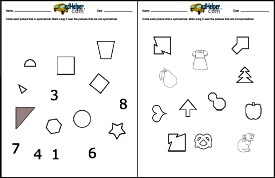Symmetry and Lines of Symmetry

Slides, Turns, and Flips

Fractions
One half - circle the shape
One half - color
One third - circle the shape
One third - color
One fourth - circle the shape
One fourth - color
What fraction does the colored part show? Circle the fraction
Color one part
Draw lines to show the fraction
Parts of a group
More parts of a group
Symmetry
Fractions and pizza

Fractions Review
Fractions Review
Fractions Review (larger review and includes pizza word problems)

Money
Counting (each problem only uses one type of coin)
Counting pennies
Counting nickels
Counting dimes
Mixed counting
Mixed counting multiple choice
Total value of coins

Nickels and Pennies
Counting
Multiple choice
Write total value (3-9 coins)
Write total value (7-12 coins)
Circle the coins that match the price
Draw a certain number of cents using a given number of coins
Fill in the missing numbers

Dimes and Pennies
Counting
Multiple choice
Write total value (3-9 coins)
Write total value (7-12 coins)
Circle the coins that match the price
Draw a certain number of cents using a given number of coins
Fill in the missing numbers

Pennies, Nickels, and Dimes
Counting
Multiple choice
Write total value (3-9 coins)
Write total value (7-12 coins)
Circle the coins that match the price
Draw a certain number of cents using a given number of coins
Fill in the missing numbers

Review
Money Review: Pennies, Nickels, and Dimes

Pennies, Nickels, and Quarters
Counting
Multiple choice
Write total value (3-9 coins)
Write total value (7-12 coins)
Circle the coins that match the price
Draw a certain number of cents using a given number of coins
Fill in the missing numbers

Pennies, Nickels, Dimes, and Quarters
Counting
Multiple choice
Write total value (3-9 coins)
Write total value (7-12 coins)
Circle the coins that match the price
Draw a certain number of cents using a given number of coins
Fill in the missing numbers

Review
Money Review: Pennies, Nickels, Dimes, and Quarters

Length and Weight
Length (black and white graphics)
Circle the object with the longest side
Number the objects in order from shortest to longest
Number the objects in order from longest to shortest
Estimate (inches and feet)
Estimate (centimeters and meters)
Weight (black and white graphics)
Circle the object that is heavier
Circle the object that is lighter
Number the objects in order from lightest to heaviest
Number the objects in order from heaviest to lightest
Estimate (ounces and pounds)
Estimate (grams and kilograms)
Review (black and white graphics)
Length and weight review (black and white graphics)

Length (color graphics)
Circle the object with the longest side
Number the objects in order from shortest to longest
Number the objects in order from longest to shortest
Estimate (inches and feet)
Estimate (centimeters and meters)
Weight (color graphics)
Circle the object that is heavier
Circle the object that is lighter
Number the objects in order from lightest to heaviest
Number the objects in order from heaviest to lightest
Estimate (ounces and pounds)
Estimate (grams and kilograms)
Review (color graphics)
Length and weight review (color graphics)

Capacity
Order Capacity: Order the objects from smallest capacity to greatest capacity
Order Capacity: Order the objects from greatest capacity to smallest capacity
Circle the container that holds more
Circle the container that holds less

Cups and Pints
Circle the set which holds more
Circle the set which holds less
Which holds more? (more difficult)
Which holds less? (more difficult)

Pints and Quarts
Circle the set which holds more
Circle the set which holds less
Which holds more? (more difficult)
Which holds less? (more difficult)

Cups and Quarts
Circle the set which holds more
Circle the set which holds less
Which holds more? (more difficult)
Which holds less? (more difficult)

Cups, Pints, and Quarts
Circle the set which holds more
Circle the set which holds less
Which holds more? (more difficult)
Which holds less? (more difficult)

Quarts and Gallons
Circle the set which holds more
Circle the set which holds less
Which holds more? (more difficult)
Which holds less? (more difficult)

Pints, Quarts, and Gallons
Circle the set which holds more
Circle the set which holds less
Which holds more? (more difficult)
Which holds less? (more difficult)

Mix
Cups and pints
Cups, pints, and quarts
Cups, pints, quarts, and gallons

Temperature
Is the temperature hot or cold in the picture?
Color the thermometer to show the temperature
Color the thermometer to show the temperature

Capacity Review
Capacity mixed review

Place Value - Numbers to 1,000
Write the number of hundreds and tens (includes blocks)
Write the number of hundreds, tens, and ones (includes blocks)
Write the number of hundreds, tens, and ones
Write the number of hundreds, tens, and ones
Write the number (100 + 30 + 2, same order used)
Write the number (with mixed order: 30 + 100 + 2)
Write the number (3 hundreds + 2 ones + 4 tens)
Number words: Write the number (one hundred fifty-seven)
Fill in the missing number (____ + 70 + 3 = 573)
Circle the value of the underlined digit
Number words: Write the number
Number words: Circle the correct way to write the number
Circle the correct ways to show each number (includes blocks)
Circle the correct ways to show each number
Place Value - Numbers to 1,000 Mixed Review

More Fractions
Write a fraction to show how much of the shape is shaded
Write a fraction to show how much of the shape is not shaded
Draw a picture to show the fraction
Same numerators
Same denominators
Either the same numerators or the same denominators
Fraction Comparison (with graphics, but not shaded in)
Same numerators
Same denominators
Fill in the missing number to make two equivalent fractions
Fill in one number: Larger graphics with fractions shown
Fill in one number: Smaller graphics with only boxes
Fill in two number: Larger graphics with fractions shown
Fill in two number: Smaller graphics with only boxes
Mixed Numbers and Improper Fractions
Multiple choice: Circle the correct improper fraction for the parts that are shaded
Multiple choice: Circle the correct mixed number for the parts that are shaded
Write an improper fraction for the parts that are shaded
Write a mixed number for the parts that are shaded
Problem Solving
Single step
Two steps
Mixed Review
Understand Fractions Mixed Review

Comparing and Ordering Numbers to 1,000
Comparing 3-Digit numbers
Number patterns: Counting by one (3 numbers and 1 missing number)
Number patterns: Counting by one (larger problems and multiple missing numbers)
Order 3 numbers from smallest to largest
Order 3 numbers from largest to smallest
Order 4 numbers from smallest to largest
Order 4 numbers from largest to smallest
Order 4 numbers from smallest to largest (number range is smaller)
Order 4 numbers from largest to smallest (number range is smaller)
Number patterns: Skip count by 2's
Number patterns: Skip count by 3's
Number patterns: Skip count by 5's
Number patterns: Skip count by 10's
Number patterns (mixed)
Comparing and Ordering Numbers to 1,000 Mixed Review

3-digit and 2-digit addition with (all are regrouping)
3-digit and 2-digit addition (most are regrouping)
Fill in the missing digit
Add three numbers (one 3-digit, two 2-digit numbers)

3-Digit Subtraction
2-Digits from 3-Digits
Subtract and then add to check
Subtract
Subtract (column headers, but no regrouping boxes)
Fill in the missing numbers
Subtract money
3-Digits
Subtract and then add to check
Subtract money
3-digit subtraction
Fill in the missing numbers
3-digit Subtraction Mixed Review

3-digit and 2-digit addition and subtraction (most are regrouping)
Fill in the missing numbers (most are regrouping)
Horizontal 2-digit and 1-digit addition and subtraction (most are regrouping)
3-digit addition and subtraction (all are regrouping)
3-digit addition and subtraction (most are regrouping)
3-digit addition and subtraction of money
Problem solving: Money word problems
Problem solving
3-digit Addition and Subtraction Mixed Review

Introduction to Multiplication
Multiplication Concepts (with graphics of pictures)
Write the sum and product
Write the addition and multiplication fact
Write the sum and product (smaller graphics)
Write the addition and multiplication fact (smaller graphics)
Multiplication with Rows (dot graphics)
Multiply with 2
Multiply with 3
Multiply with 4
Multiply with 5
Multiply with 6
Mixed
Write the sum and then write the product
Multiply with 2
Multiply with 3
Multiply with 4
Multiply with 5
Multiply with 6
Multiply with 7
Multiply with 8
Multiply with 9
Multiply with 10
Mixed
Addition and Multiplication: Fill in the missing numbers
Multiply with 2
Multiply with 3
Multiply with 4
Multiply with 5
Multiply with 6
Multiply with 7
Multiply with 8
Multiply with 9
Multiply with 10
Mixed
Comparison
Introduction to Multiplication: Mixed Review
Introduction to Multiplication Mixed Review

Introduction to Division
Division Concepts (with graphics of pictures)
Divide into groups (smaller numbers)
Divide into groups (larger numbers)
Divide into groups (mix)
Division Concepts with left over graphics
Divide into groups (smaller numbers)
Divide into groups (larger numbers)
Divide into groups (mix)
Division Facts (with graphics)
Write a division fact (smaller numbers)
Write a division fact (larger numbers)
Write a division fact (mix)
Multiplication and Division
Fill in the quotient
Fill in the missing number
Fill in the missing number